# GRE Math : How to find the solution to an inequality with multiplication

## Example Questions

### Example Question #15 : Inequalities

Quantitative Comparison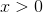Column A: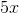Column B: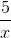Quantity B is greater.

The quantities are equal.

Quantity A is greater.

The relationship cannot be determined from the information provided.

The relationship cannot be determined from the information provided.

Explanation:

For quantitative comparison questions involving a shared variable between quantities, the best approach is to test a positive integer, a negative integer, and a fraction. Half of our work is eliminated, however, because the question stipulates that x > 0.  We only need to check a positive integer and a positive fraction between 0 and 1. Plugging in 2, we see that quantity A is greater than quantity B. Checking 1/2, however, we find that quantity B is greater than quantity A. Thus the relationship cannot be determined.

### Example Question #171 : Equations / Inequalities

If –1 < n < 1, all of the following could be true EXCEPT:

(n-1)2 > n

16n2 - 1 = 0

n2 < 2n

n2 < n

|n2 - 1| > 1

|n2 - 1| > 1

Explanation: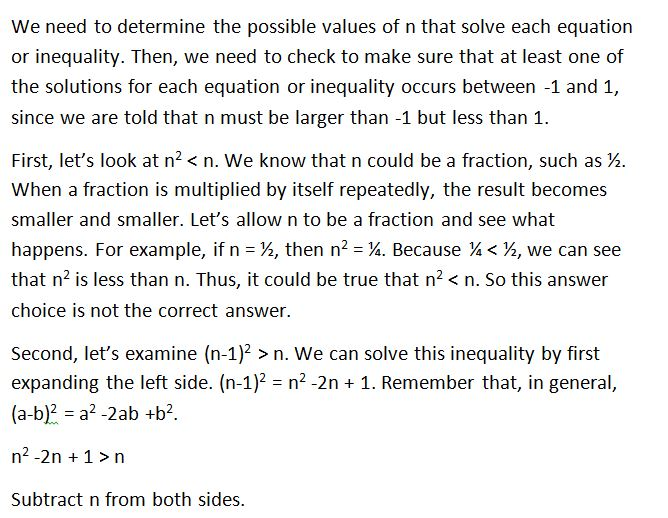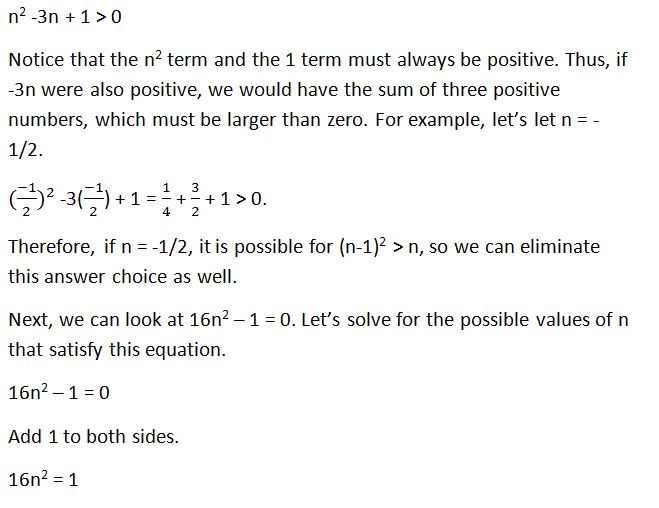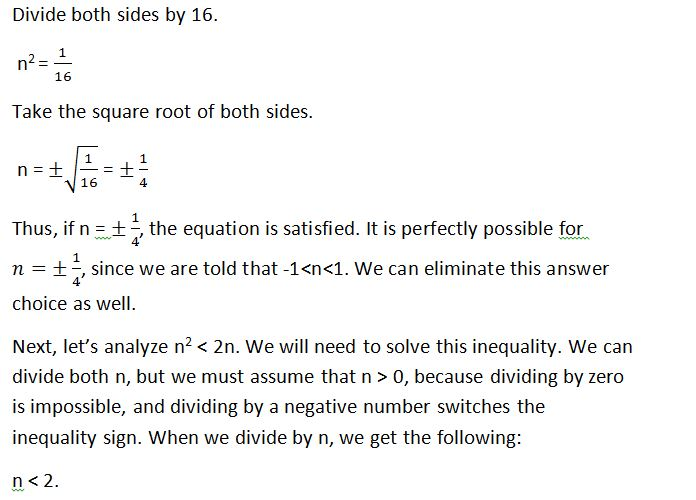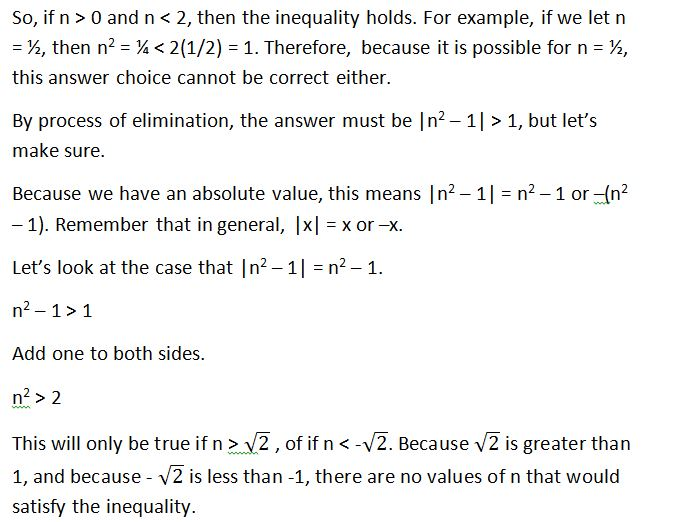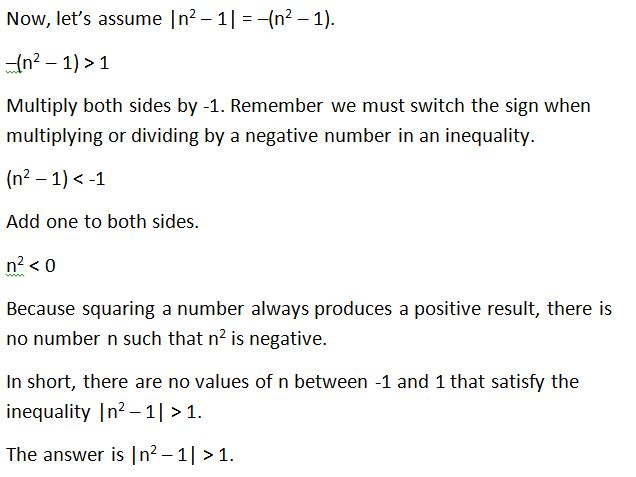### Example Question #32 : Inequalities

(√(8) / -x ) <  2. Which of the following values could be x?

All of the answers choices are valid.

-3

-1

-2

-4

-1

Explanation:

The equation simplifies to x > -1.41. -1 is the answer.

### Example Question #33 : Inequalities

Solve for x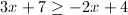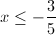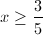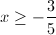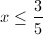Explanation: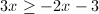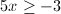### Example Question #34 : Inequalities

We have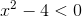, find the solution set for this inequality.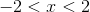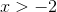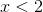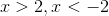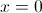Explanation: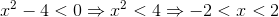### Example Question #31 : Inequalities

Fill in the circle with either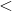,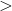, orsymbols: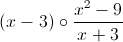for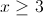.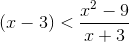The rational expression is undefined.

None of the other answers are correct.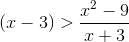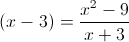Explanation:Let us simplify the second expression. We know that: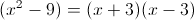So we can cancel out as follows: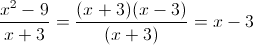### Example Question #221 : Algebra

Solve the inequality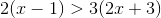.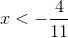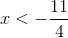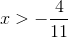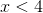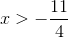Explanation:

Start by simplifying the expression by distributing through the parentheses to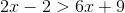.

Subtractfrom both sides to get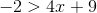.

Next subtract 9 from both sides to get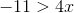. Then divide by 4 to get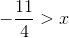which is the same as.

### Example Question #222 : Algebra

Solve the inequality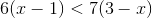.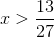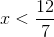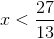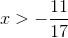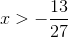Explanation:

Start by simplifying each side of the inequality by distributing through the parentheses.

This gives us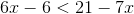.

Add 6 to both sides to get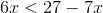.

Add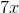to both sides to get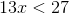.

Divide both sides by 13 to get.

Tired of practice problems?

Try live online GRE prep today.